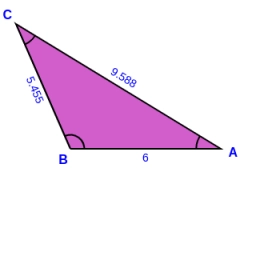# Calculate 81757

Calculate the size of the largest angle in triangle ABC if a = 7 cm, b = 8 cm, and c = 13 cm. Calculate the area of the triangle, the height per side a.

S =  24.2487 cm2
v =  6.9282 cm

### Step-by-step explanation:Did you find an error or inaccuracy? Feel free to write us. Thank you!

Tips for related online calculators